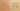RecursiveMethodImpliesRecursiveType

Misconception:

If a class C contains a recursive method m(), then class C is part of a recursive data structure.Incorrect

A class with a recursive method represents part of a recursive data structure

Correct

A class having a recursive method does not imply that it is part of a recursive data structure

CorrectionHere is what's right.

To be part of a recursive data structure, there needs to be a recursive dependency (a cycle) through the fields (instance variables) of the class.

A value of that class needs to (directly or indirectly) refer to a value of that class.

Class R below represents a recursive data structure (but has no recursive method):

class R {
R f;
}

Class N below is not part of a recursive data structure (even though it contains the recursive method sum):

class N {
int sum(int n) {
return n==0 ? 0 : 1 + sum(n - 1);
}
}

ValueHow can you build on this misconception?

Seeing recursive data structures and recursive computation as closely related is actually a pretty good idea.

Recursive data structures and recursive methods go together quite well. This “collaboration” leads to structural recursion: a recursive method traverses a recursive data structure, and the recursive computation is driven by the recursive data structure. Common examples of structural recursion are traversal algorithms for lists (e.g., add up elements in a linked list) or trees (e.g., find a value in a binary tree).

Structural recursion is simpler than generative recursion, where the recursive computation is not driven by a recursive data structure. Common examples of generative recursion are the Fibonacci sequence, factorial, or the Towers of Hanoi.